# Aligning adult mouse coronal brain sections with the Allen Brain Altas

We will use STalign to achieve this alignment. We will first load the relevant code libraries.

:

#Import dependencies

import numpy as np
%matplotlib notebook
import matplotlib.pyplot as plt
import pandas as pd # for csv.
from matplotlib import cm
from matplotlib.lines import Line2D
import os
from os.path import exists,split,join,splitext
from os import makedirs
import glob
import requests
from collections import defaultdict
import nrrd
import torch
from torch.nn.functional import grid_sample
from STalign import STalign
import copy
import skimage
from mpl_toolkits.mplot3d.art3d import Poly3DCollection
import pandas as pd


Now, we can read in the cell infomation using pandas as pd.

We must load all cell x and y positions into the variable x and y respectively. Importantly, the scale of the loaded data must approximately match the Allen Brain Atlas, where ~1 pixel unit is ~1 um

:

#Load file

filename = '__'

x = np.array(df['center_x']) #change to x positions of cells

y = np.array(df['center_y']) #change to column y positions of cells

:

dx=10
blur = 1


Now we can load in data from the Allen Brain Altas.

We need to load in and save files containing the ontology of regions, a 3D cell density atlas, and annotations of brain regions in the aforementioned 3D atlas.

Depending on which Allen Atlas you would like to use, you can change the url that you download these files from. In this example, we are aligning the adult mouse (P56) Allen Atlas.

:

url = 'http://api.brain-map.org/api/v2/data/query.csv?criteria=model::Structure,rma::criteria,[ontology_id$eq1],rma::options[order$eq%27structures.graph_order%27][num_rows\$eqall]'


<Response >

:

imageurl = 'http://download.alleninstitute.org/informatics-archive/current-release/mouse_ccf/ara_nissl/ara_nissl_50.nrrd'


Our first step in this alignment will be to rasterize the single cell centroid positions into an image. This converts our x and y arrays of cells into a pixelated image. For this example, we choose to rasterize at a resolution of 15 (um).

:

#Rasterize Image
X_,Y_,W = STalign.rasterize(x,y,dx=dx, blur = blur,draw=False)


We can visualize the resulting rasterized image.

:

#Plot unrasterized/rasterized images
fig,ax = plt.subplots(1,2)
ax.scatter(x,y,s=0.5,alpha=0.25)
ax.invert_yaxis()
ax.set_title('List of cells')
ax.set_aspect('equal')

W = W
extent = (X_,X_[-1],Y_,Y_[-1])
ax.imshow(W,  origin='lower')
ax.invert_yaxis()
ax.set_title('Rasterized')

# save figure
#fig.canvas.draw()
#fig.savefig(outname[:-4]+'_image.png')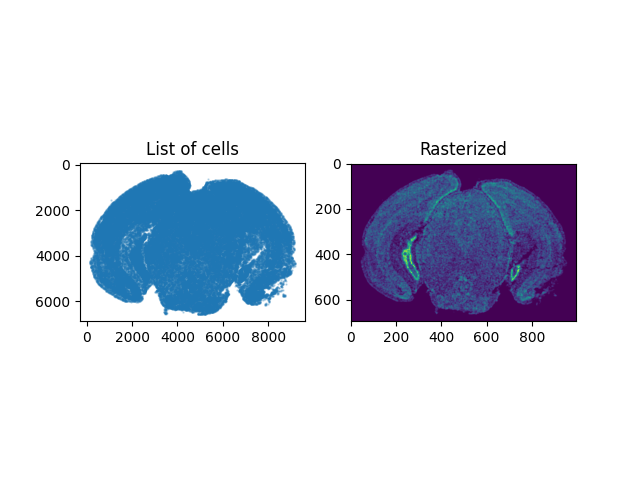:

Text(0.5, 1.0, 'Rasterized')


Now we need to find an approximate slice number in the Allen Atlas to initialize the alignment. Evaluate whether the value of slice is similar to the target image by viewing a side by side comparison of the ABA slice and the target image. If not, change the value of slice. In this example, slice = 0 is anterior and slice = 264 is posterior.

:

#find slice
#peruse through images in atlas
slice = 177

A = vol
L = vol

dxA = np.diag(hdr['space directions'])
nxA = A.shape
xA = [np.arange(n)*d - (n-1)*d/2.0 for n,d in zip(nxA,dxA)]
XA = np.meshgrid(*xA,indexing='ij')

fig,ax = plt.subplots(1,2)
extentA = STalign.extent_from_x(xA[1:])
ax.imshow(A[slice],extent=extentA)
ax.set_title('Atlas Slice')

ax.imshow(W,extent=extentA)
ax.set_title('Target Image')
fig.canvas.draw()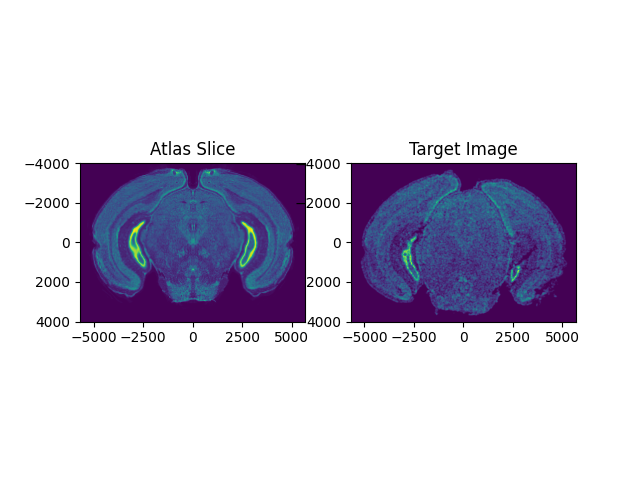Next, we need to find an approximate rotation angle of the Allen Atlas to initialize the alignment. Evaluate whether the rotation of the atlas is similar to the target image by viewing a side by side comparison of the ABA slice and the target image. If not, change the value of theta_deg. Note: the rotation here is defined in degrees.

:

from scipy.ndimage import rotate

theta_deg = 0

fig,ax = plt.subplots(1,2)
extentA = STalign.extent_from_x(xA[1:])
ax.imshow(rotate(A[slice], angle=theta_deg),extent=extentA)
ax.set_title('Atlas Slice')

ax.imshow(W,extent=extentA)
ax.set_title('Target Image')
fig.canvas.draw()Next, specify if there are any points on the image above that can be approximately matched with eachother and add them in the following format. This helps speed up alignment. (OPTIONAL)

:

points_atlas = np.array([[0,2580]])
points_target = np.array([[8,2533]])
Li,Ti = STalign.L_T_from_points(points_atlas,points_target)


We can define atlas and target points, xI and xJ, as well as atlas and target images, I and J.

:

xJ = [Y_,X_]
J = W[None]/np.mean(np.abs(W))
xI = xA
I = A[None] / np.mean(np.abs(A),keepdims=True)
I = np.concatenate((I,(I-np.mean(I))**2))


Now that we can chosen the slice number and rotation angle, we are ready to initialize parameters related to our alignment.

:

sigmaA = 2 #standard deviation of artifact intensities
sigmaB = 2 #standard deviation of background intensities
sigmaM = 2 #standard deviation of matching tissue intenities
muA = torch.tensor([3,3,3],device='cpu') #average of artifact intensities
muB = torch.tensor([0,0,0],device='cpu') #average of background intensities


We can change the parameters above by looking at the intensity histogram of our target image (below). We need to consider intensities of artifacts (tissue that is present in the target image and absent in the atlas), which is usually in the upper range of intensity values. We also need to find the intensity of the background values that does not correspond to any tissue, usually around 0, and standard deviations for these values. The matching tissue intensities are regions that should have tissue that can be aligned in both the atlas and the target image.

:

fig,ax = plt.subplots()
ax.hist(J.ravel())
plt.xlabel('Intensity')
plt.ylabel('Number of Pixels')
plt.title('Intensity Histogram of Target Image')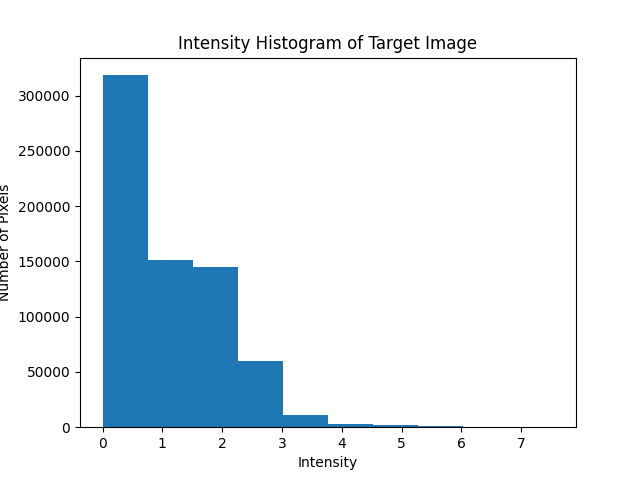:

Text(0.5, 1.0, 'Intensity Histogram of Target Image')


The following parameters vary depending on your target image and the duration & accuracy of the alignment. The scale parameters refer to a scaling of the atlas in (x, y, z) to match the size of the target image. The nt parameter ensures smoothness and invertibility of the image transformation. The niter refers to the number of iteration steps in gradient descent to achieve minimum error between the altas and the target image.

:

# initialize variables
scale_x = 0.9 #default = 0.9
scale_y = 0.9 #default = 0.9
scale_z = 0.9 #default = 0.9
theta0 = (np.pi/180)*theta_deg

# get an initial guess
if 'Ti' in locals():
T = np.array([-xI[slice],np.mean(xJ)-(Ti*scale_y),np.mean(xJ)-(Ti*scale_x)])
else:
T = np.array([-xI[slice],np.mean(xJ),np.mean(xJ)])

scale_atlas = np.array([[scale_z,0,0],
[0,scale_x,0],
[0,0,scale_y]])
L = np.array([[1.0,0.0,0.0],
[0.0,np.cos(theta0),-np.sin(theta0)],
[0.0,np.sin(theta0),np.cos(theta0)]])
L = np.matmul(L,scale_atlas)#np.identity(3)


Now, we can perform alignment of the atlas to our target slice.

:

%%time

# run LDDMM
# specify device (default device for STalign.LDDMM is cpu)
if torch.cuda.is_available():
device = 'cuda:0'
else:
device = 'cpu'

#returns mat = affine transform, v = velocity, xv = pixel locations of velocity points
transform = STalign.LDDMM_3D_to_slice(
xI,I,xJ,J,
T=T,L=L,
nt=4,niter=2000,
device='cpu',
sigmaA = sigmaA, #standard deviation of artifact intensities
sigmaB = sigmaB, #standard deviation of background intensities
sigmaM = sigmaM, #standard deviation of matching tissue intenities
muA = muA, #average of artifact intensities
muB = muB #average of background intensities
)

/Users/gohtaaihara/.local/share/virtualenvs/STalign-oDkYRjeO/lib/python3.11/site-packages/torch/functional.py:504: UserWarning: torch.meshgrid: in an upcoming release, it will be required to pass the indexing argument. (Triggered internally at /Users/runner/work/pytorch/pytorch/pytorch/aten/src/ATen/native/TensorShape.cpp:3484.)
return _VF.meshgrid(tensors, **kwargs)  # type: ignore[attr-defined]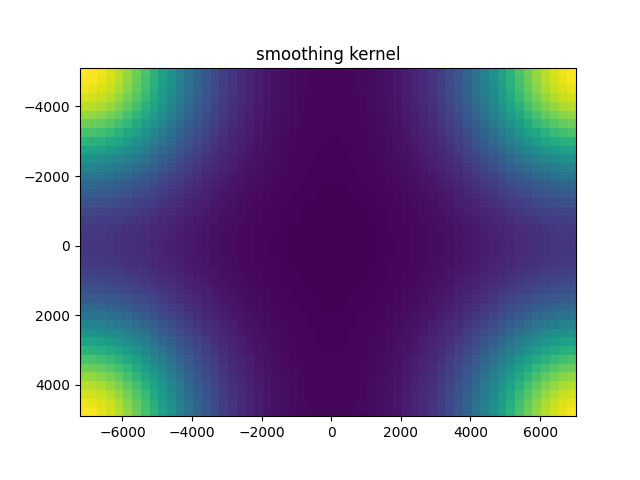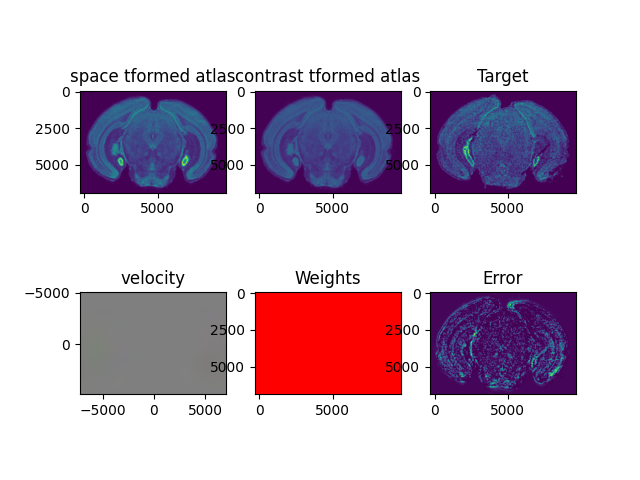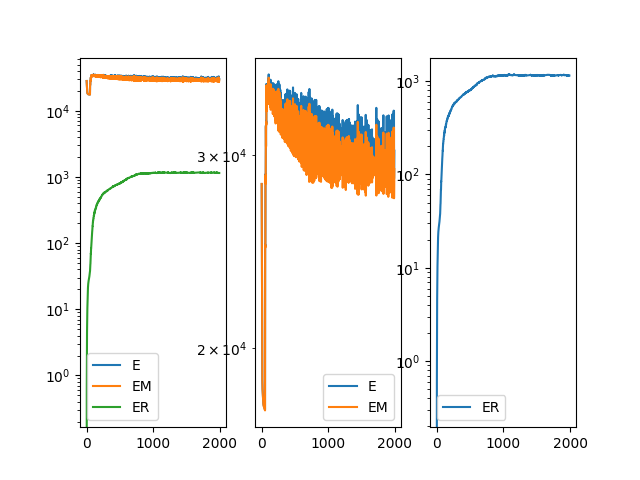/Users/gohtaaihara/.local/share/virtualenvs/STalign-oDkYRjeO/lib/python3.11/site-packages/STalign/STalign.py:1616: UserWarning: Data has no positive values, and therefore cannot be log-scaled.
axE.set_yscale('log')

CPU times: user 3h 24min 29s, sys: 21min 43s, total: 3h 46min 13s
Wall time: 1h 27min 45s

:

A = transform['A']
v = transform['v']
xv = transform['xv']
Xs = transform['Xs']


Once we have aligned the atlas to our target image, we can project the brain regions defined in the atlas to our target image and export these annotations, along with information about the positions of the atlas that align with the slice.

:

df = STalign.analyze3Dalign(labelfile,  xv,v,A, xJ, dx, scale_x=scale_x, scale_y=scale_y,x=x,y=y, X_=X_, Y_=Y_, namesdict=namesdict,device='cpu')

/Users/gohtaaihara/.local/share/virtualenvs/STalign-oDkYRjeO/lib/python3.11/site-packages/STalign/STalign.py:1725: UserWarning: To copy construct from a tensor, it is recommended to use sourceTensor.clone().detach() or sourceTensor.clone().detach().requires_grad_(True), rather than torch.tensor(sourceTensor).
A = torch.tensor(A)
/Users/gohtaaihara/.local/share/virtualenvs/STalign-oDkYRjeO/lib/python3.11/site-packages/STalign/STalign.py:1726: UserWarning: To copy construct from a tensor, it is recommended to use sourceTensor.clone().detach() or sourceTensor.clone().detach().requires_grad_(True), rather than torch.tensor(sourceTensor).
v = torch.tensor(v)
/Users/gohtaaihara/.local/share/virtualenvs/STalign-oDkYRjeO/lib/python3.11/site-packages/STalign/STalign.py:1738: UserWarning: To copy construct from a tensor, it is recommended to use sourceTensor.clone().detach() or sourceTensor.clone().detach().requires_grad_(True), rather than torch.tensor(sourceTensor).
XJ = torch.tensor(XJ)

:

df

:

coord0 coord1 coord2 x y struct_id acronym
0 2413.110401 569.983430 -5212.124371 156.563284 4271.326432 0 bg
1 2412.983413 558.903980 -5212.634045 156.509284 4256.962431 0 bg
2 2412.604358 525.665751 -5214.161392 159.965284 4228.180431 0 bg
3 2413.760514 635.721790 -5196.641113 167.579284 4323.868433 0 bg
4 2413.621536 614.301429 -5210.082881 160.559284 4308.802433 0 bg
... ... ... ... ... ... ... ...
78324 2399.125365 521.339869 5193.726908 9154.007886 4445.528506 0 bg
78325 2397.838721 499.764931 5121.432239 9088.829884 4423.712505 0 bg
78326 2399.007551 498.304093 5214.957544 9170.261887 4431.758506 0 bg
78327 2397.635522 488.530178 5120.404084 9086.183884 4417.016505 0 bg
78328 2397.346986 488.642219 5096.902566 9073.169884 4419.770505 0 bg

78329 rows × 7 columns

Now, we can explore our alignment and brain region annotations!

We can visualize the MERFISH slice overlayed with the matched section in the Allen Brain Atlas.

:

It = torch.tensor(I,device='cpu',dtype=torch.float64)
Ishow_source = ((AI-torch.amin(AI,(1,2,3))[...,None,None])/(torch.amax(AI,(1,2,3))-torch.amin(AI,(1,2,3)))[...,None,None,None]).permute(1,2,3,0).clone().detach().cpu()
Jt = torch.tensor(J,device='cpu',dtype=torch.float64)
Ishow_target = Jt.permute(1,2,0).cpu()/torch.max(Jt).item()

import matplotlib as mpl
fig,ax = plt.subplots(1,3, figsize=(15,5))
ax0 = ax.imshow(Ishow_target, cmap = mpl.cm.Blues,alpha=0.9)
ax.set_title('MERFISH Slice')
ax1 = ax.imshow(Ishow_source[0,:,:,0], cmap = mpl.cm.Reds,alpha=0.2)
ax.set_title('z=0 slice of Aligned 3D Allen Brain Atlas')
ax2 = ax.imshow(Ishow_target, cmap = mpl.cm.Blues,alpha=0.9)
ax2 = ax.imshow(Ishow_source[0,:,:,0], cmap = mpl.cm.Reds,alpha=0.3)
ax.set_title('Overlayed')

plt.show()